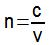Optics

# Snell-Descartes Law

The Snell-Descartes law relates the angles formed between the rays of light and the normal line with the refractive indices of the media under analysis.

Refraction is the optical phenomenon characterized by a change in the speed of light due to a change in the propagation medium.

Refractive index

The refractive index characterizes each light propagation medium, revealing the intensity of the change in the speed of the generated light waves . This dimensionless quantity is determined by the relationship between the speed of light in vacuum (c) and the speed of light in the medium under analysis (v).If a certain propagation medium has a refractive index equal to 1.5, this means that, when penetrating this medium, light will have a speed 1.5 times lower than its maximum speed in vacuum (3.0 x 10 8 m/ s).

Angles of Incidence and Refraction

The image below shows a ray of light undergoing refraction, passing from a medium with index of refraction n 1 to another medium with index n 2 .

The normal line (N) is perpendicular to the surface separating media 1 and 2. The angle θ 1 is called the angle of incidence, and is always formed between the incident light ray and the normal line. The angle θ 2 is called the angle of refraction, and it is always formed between the refracted ray of light and the normal line.

If the ray of light passes to a region of higher refractive index, there will be a decrease in the value of the velocity, and then it will approach the normal line. If the ray of light changes to a region of lower refractive index, there will be an increase in the velocity value, and then it will move away from the normal line.

Proposed in 1621 by mathematician Villebrord Snell and philosopher René Descartes , the well-known Snell-Descartes law relates the angles of incidence and refraction with the refractive indices of the media involved.

This law determines that the product of the sine of the angle formed between the ray of light and the normal line and the refractive index of the medium must be constant . From the previous image, we can write this law as follows:If the ray of light passes to a region of higher refractive index, there will be a decrease in the value of the velocity, and then it will approach the normal line. If the ray of light changes to a region of lower refractive index, there will be an increase in the velocity value, and then it will move away from the normal line.

Example:

(UEFS BA) A light ray is incident on the surface separating air and glass at an angle α = 60° and refracts at an angle β = 30°, as shown in the figure.

Considering sin30º = cos60º = 0.5; sin60º = cos30º = 0.87; the refractive index of air equals 1 and the refractive index of glass equals an, then the value of n is equal to

01. 1.48

02. 1.57

03. 1.63

04. 1.74

05. 1.83